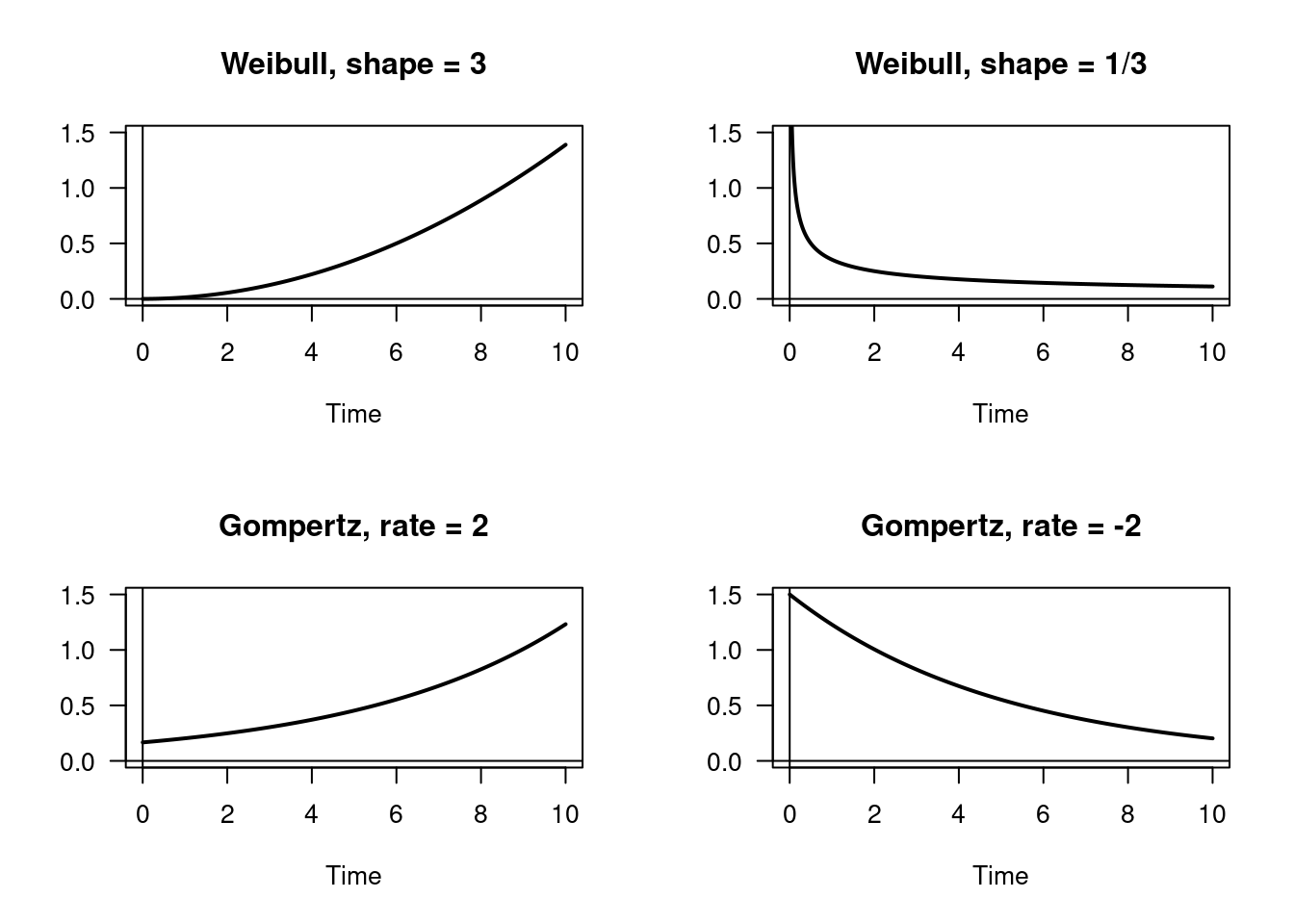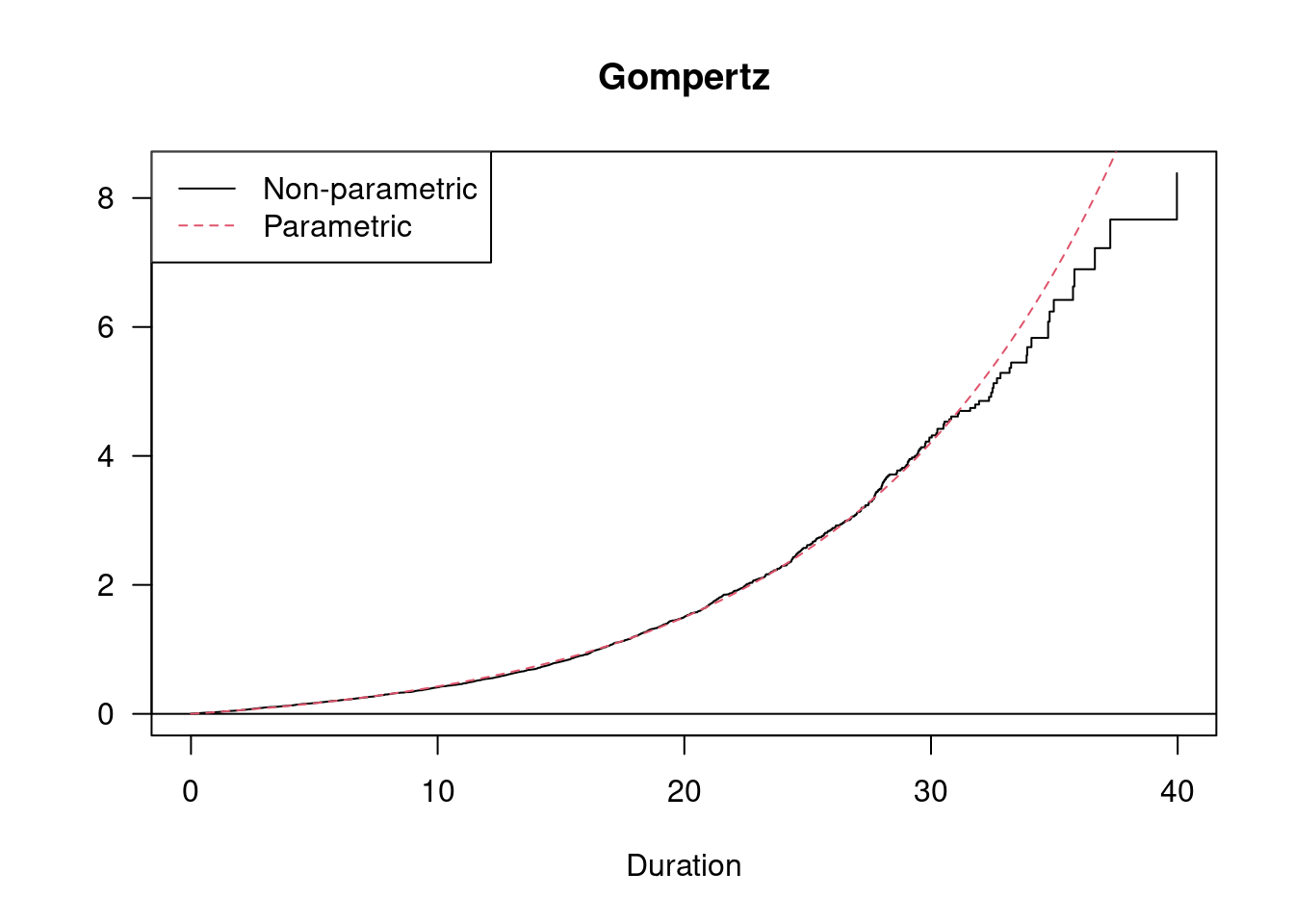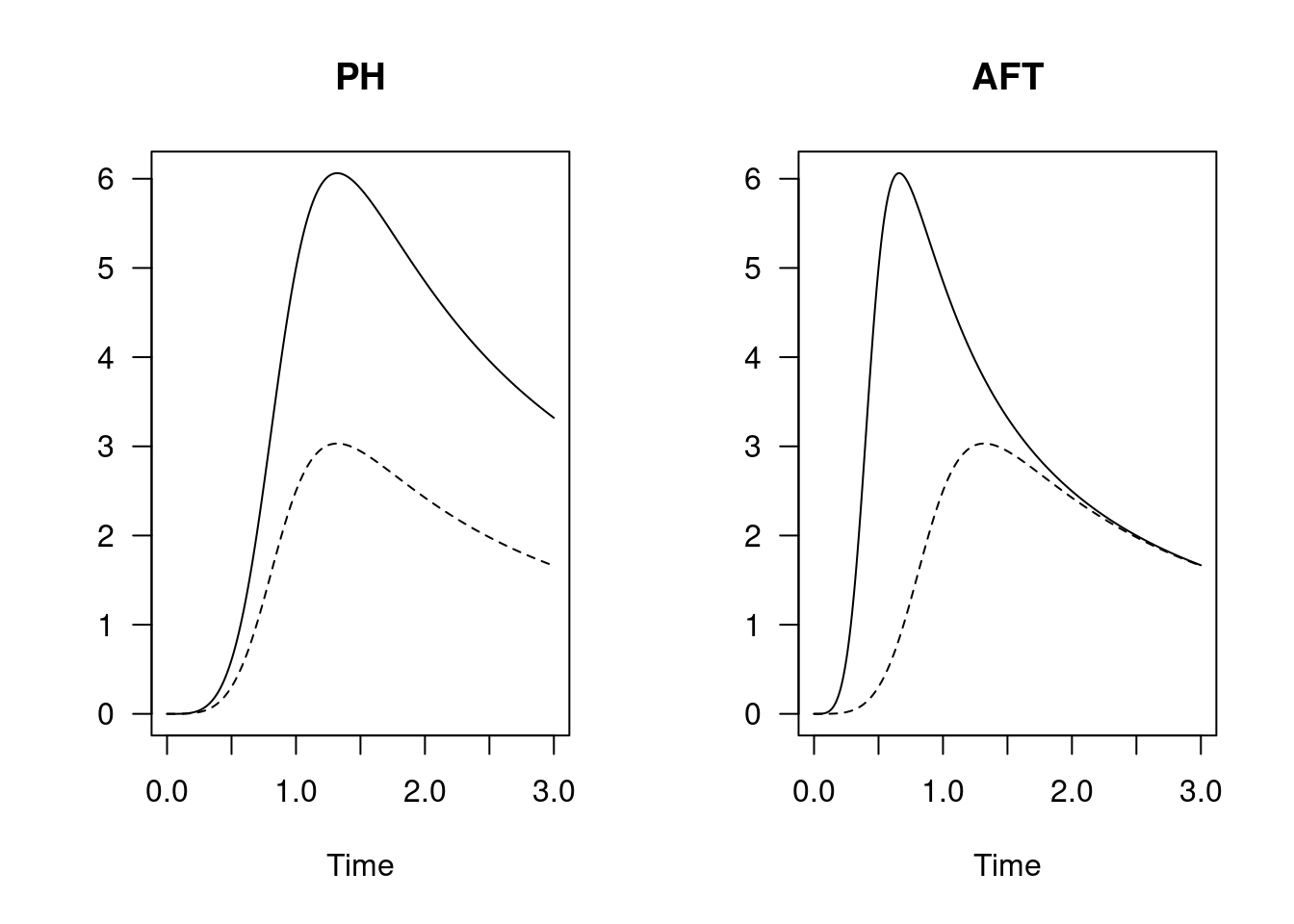A unified implementation of parametric proportional hazards (PH) and accelerated failure time (AFT) models for right-censored or interval-censored and left-truncated data is described in a separate paper. It gives the mathematical background, but the user guide is this vignette.

# 1 The proportional hazards model

The parametric proportional hazards (PH) model has the same characteristics as Cox’s proportional hazards model, with the exception that the baseline hazard function in the parametric case is explicitly estimated together with regression coefficients (if any). If two hazard functions $$h_0$$ and $$h_1$$ have the property that $\begin{equation} h_1(t) = \psi h_0(t), \quad \text{for all t > 0 and some \psi > 0}, \end{equation}$ we say that they are proportional. This property is inherited by the cumulative hazards functions:

$\begin{equation} H_1(t) = \psi H_0(t), \quad \text{for all t > 0 and some \psi > 0}, \end{equation}$ but the relation between the corresponding survivor functions becomes

$\begin{equation} S_1(t) = \{S_0(t)\}^\psi, \quad \text{for all t > 0 and some \psi > 0}. \end{equation}$

We assume here that $$\psi$$ is constant, not varying with $$t$$.

## 1.1 Available distributions

The parametric distribution functions that naturally can be used as the baseline distribution in the function phreg are the Weibull, Piecewise constant hazard (pch), Extreme value and the Gompertz distributions. The lognormal and loglogistic distributions are also included as possible choices and allow for hazard functions that are first increasing to a maximum and then decreasing, while the other distributions all have monotone hazard functions. However, since these families are not closed under proportional hazards without artificially adding a third, “proportionality”, parameter, they are not discussed here (regard these possibilities as experimental). It is better to combine the lognormal and the loglogistic distributions with the accelerated failure time modeling, where they naturally fit in.

See Figure 1.1 for Weibull and Gompertz hazard functions with different parameter values.Figure 1.1: Selected hazard functions.

We note in passing that the fourth case, the Gompertz model with negative rate parameter, does not represent a true survival distribution, because the hazard function decreases too fast: There will be a positive probability of eternal life.

## 1.2 An example, old age mortality

The data set oldmort in the R package eha contains life histories of people followed from their 60th birthday to their 100th, or until death. They are all born between June 28, 1765 and December 31, 1820 in Skellefteå.

We fit a Gompertz model:

fit.g <- phreg(Surv(enter - 60, exit - 60, event) ~ sex + civ + region,
dist = "gompertz", data = oldmort)
summary(fit.g)       
## Covariate             Mean       Coef     Rel.Risk   S.E.    LR p
## sex                                                          0.000
##             male      0.406     0         1 (reference)
##           female      0.594    -0.239     0.788     0.047
## civ                                                          0.000
##        unmarried      0.080     0         1 (reference)
##          married      0.530    -0.416     0.660     0.082
##            widow      0.390    -0.262     0.770     0.080
## region                                                       0.001
##             town      0.111     0         1 (reference)
##         industry      0.326     0.265     1.303     0.086
##            rural      0.563     0.119     1.127     0.084
##
## Events                    1971
## Total time at risk         37824
## Max. log. likelihood       -7268
## LR test statistic         56.99
## Degrees of freedom        5
## Overall p-value           5.08754e-11

Then we fit a Cox regression model.

fit.c <- coxreg(Surv(enter - 60, exit - 60, event) ~ sex + civ + region, data = oldmort)
summary(fit.c)
## Covariate             Mean       Coef     Rel.Risk   S.E.    LR p
## sex                                                          0.000
##             male      0.406     0         1 (reference)
##           female      0.594    -0.235     0.791     0.047
## civ                                                          0.000
##        unmarried      0.080     0         1 (reference)
##          married      0.530    -0.410     0.664     0.082
##            widow      0.390    -0.262     0.770     0.080
## region                                                       0.001
##             town      0.111     0         1 (reference)
##         industry      0.326     0.268     1.308     0.086
##            rural      0.563     0.122     1.130     0.084
##
## Events                    1971
## Total time at risk         37824
## Max. log. likelihood      -13551
## LR test statistic         55.71
## Degrees of freedom        5
## Overall p-value           9.32227e-11

And we compare the estimated baseline hazards.

check.dist(fit.c, fit.g)The fit of the Gompertz baseline function is very close to the non-parametric one, indicating that old age mortality is exponentially increasing with age. However, in the last ten years or so (ages 90+), the increase seems to slow down.

# 2 The accelerated failure time model

The accelerated failure time (AFT) model is best described through relations between survivor functions. For instance, comparing two groups:

• Group 0: $$P(T \ge t) = S_0(t)$$ (control group)
• Group 1: $$P(T \ge t) = S_0(\phi t)$$ (treatment group)

The model says that treatment accelerates} failure time by the factor $$\phi$$. If $$\phi < 1$$, treatment is good (prolongs life), otherwise bad. Another interpretation is that the median* life length is multiplied by $$1/\phi$$ by treatment.

In Figure 2.1 the difference between the accelerated failure time and the proportional hazards models concerning the hazard functions is illustrated.Figure 2.1: Proportional hazards (left) and accelerated failure time model (right). The baseline distribution is Loglogistic with shape 5 (dashed).

The AFT hazard is not only multiplied by 2, it is also shifted to the left; the process is accelerated. Note how the hazards in the AFT case converges as time increases. This is usually a sign of the suitability of an AFT model.

If $$T$$ has survivor function $$S(t)$$ and $$T_c = T/c$$, then $$T_c$$ has survivor function $$S(ct)$$. Then, if $$Y = \log(T)$$ and $$Y_c = \log(T_c)$$, the following relation holds:

$\begin{equation*} Y_c = Y - log(c). \end{equation*}$

With $$Y = \epsilon$$, $$Y_c = Y$$, and $$\log(c) = -\boldsymbol{\beta} \mathbf{x}$$ this can be written in familiar form:

$\begin{equation*} Y = \boldsymbol{\beta} \mathbf{x} + \epsilon, \end{equation*}$

That is, an ordinary linear regression model for the log survival times. In the absence of right censoring and left truncation, this model can be estimated by least squares. However, the presence of these forms of incomplete data makes it necessary to rely on maximum likelihood methods. In R, there is the functions aftreg in the package eha and the function survreg in the package survival that perform the task of fitting AFT models.

Besides differing parametrizations, the main difference between aftreg and survreg is that the latter does not allow for left truncated data. One reason for this is that left truncation is a much harder problem to deal with in AFT models than in proportional hazards models.

## 2.1 Available distributions

In aftreg the available baseline distributions are the Gompertz, Weibull, Extreme value, Lognormal, and Loglogistic distributions.

## 2.2 The oldmort data

We repeat the analysis of the old age mortality data, but with an accelerated failure time model.

fit.g1 <- aftreg(Surv(enter - 60, exit - 60, event) ~ sex + civ + region, data = oldmort, dist = "gompertz")
summary(fit.g1)
## Covariate             Mean       Coef     Rel.Risk   S.E.    LR p
## sex                                                          0.000
##             male      0.406     0         1 (reference)
##           female      0.594    -0.078     0.925     0.020
## civ                                                          0.000
##        unmarried      0.080     0         1 (reference)
##          married      0.530    -0.159     0.853     0.035
##            widow      0.390    -0.100     0.905     0.032
## region                                                       0.007
##             town      0.111     0         1 (reference)
##         industry      0.326     0.111     1.117     0.040
##            rural      0.563     0.071     1.073     0.040
##
## Events                    1971
## Total time at risk         37824
## Max. log. likelihood      -7276.3
## LR test statistic         40.29
## Degrees of freedom        5
## Overall p-value           1.30748e-07

# 3 Proportional hazards or AFT model?

The problem of choosing between a proportional hazards and an accelerated failure time model (everything else equal) can be solved by comparing the AIC of the models. Since the numbers of parameters are equal in the two cases, this amounts to comparing the maximized likelihoods. For instance, in the case with old age mortality:

Comparing the corresponding result for the proportional hazards and the AFT models with the Gompertz distribution, we find that the maximized log likelihood in the latter case is -7276.314, compared to -7267.963 for the former. This indicates that the proportional hazards model fit is better. Note however that we cannot formally test the proportional hazards hypothesis; the two models are not nested.